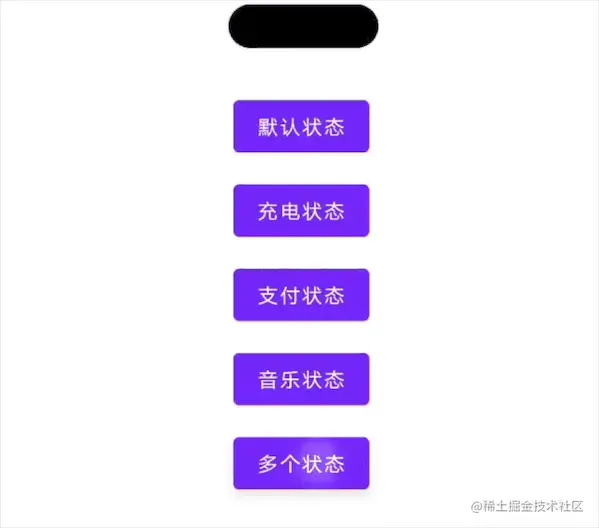# Compose 动画艺术探索之灵动岛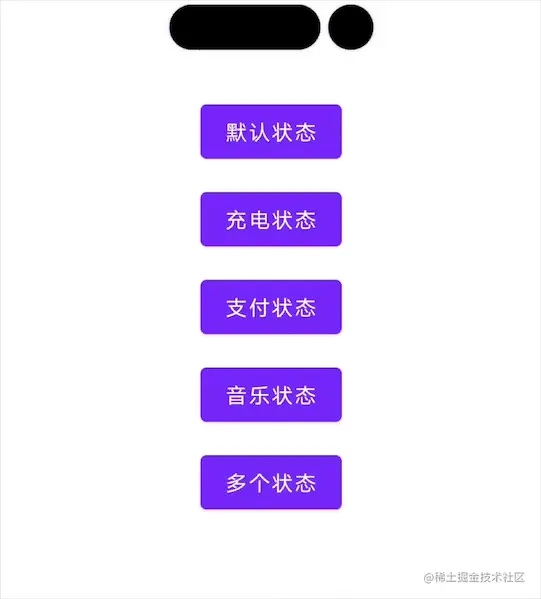## 苹果的灵动岛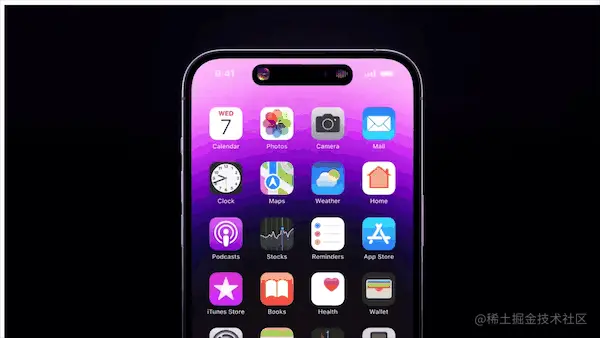## Compose 简单实现

``````@Composable
fun DynamicScreen() {
var isCharge by remember { mutableStateOf(true) }
​
val animateSizeAsState by animateSizeAsState(
targetValue = Size(if (isCharge) 170f else 100f, 30f)
)
​
Column {
Box(modifier = Modifier
.width(animateSizeAsState.width.dp)
.height(animateSizeAsState.height.dp)
.shadow(elevation = 3.dp, shape = RoundedCornerShape(15.dp))
.background(color = Color.Black),
)
​
Button(
modifier = Modifier.padding(top = 30.dp, bottom = 5.dp),
onClick = { isCharge = false }) {
Text(text = "默认状态")
}
​
Button(
onClick = { isCharge = true }) {
Text(text = "充电状态")
}
}
}
``````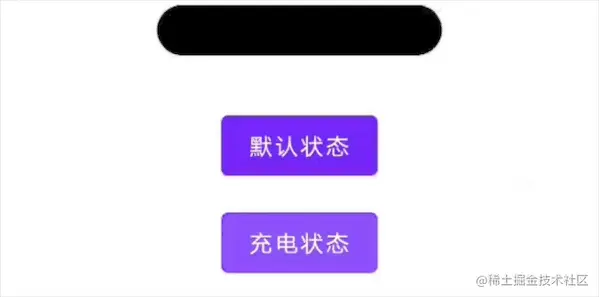``````val animateSizeAsState by animateSizeAsState(
targetValue = Size(if (isCharge) 170f else 100f, 30f),
animationSpec = spring(
dampingRatio = Spring.DampingRatioLowBouncy,
stiffness = Spring.StiffnessMediumLow
)
)
``````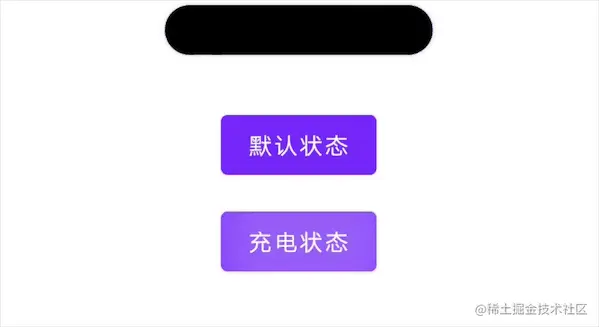## 实现多种切换

``````private sealed class BoxState(val height: Dp, val width: Dp) {
// 默认状态
object NormalState : BoxState(30.dp, 100.dp)
​
// 充电状态
object ChargeState : BoxState(30.dp, 170.dp)
​
// 支付状态
object PayState : BoxState(100.dp, 100.dp)
​
// 音乐状态
object MusicState : BoxState(170.dp, 340.dp)
​
// 多个状态
object MoreState : BoxState(30.dp, 100.dp)
}
``````

``````var boxState: BoxState by remember { mutableStateOf(BoxState.NormalState) }
``````

``````val animateSizeAsState by animateSizeAsState(
targetValue = Size(boxState.width.value, boxState.height.value),
animationSpec = spring(
dampingRatio = Spring.DampingRatioLowBouncy,
stiffness = Spring.StiffnessMediumLow
)
)
``````

``````Button(
modifier = Modifier.padding(top = 30.dp, bottom = 5.dp),
onClick = { boxState = BoxState.NormalState }) {
Text(text = "默认状态")
}
​
Button(
onClick = { boxState = BoxState.ChargeState }) {
Text(text = "充电状态")
}
``````

``````Button(
onClick = { boxState = BoxState.PayState }) {
Text(text = "支付状态")
}
​
Button(
onClick = { boxState = BoxState.MusicState }) {
Text(text = "音乐状态")
}
``````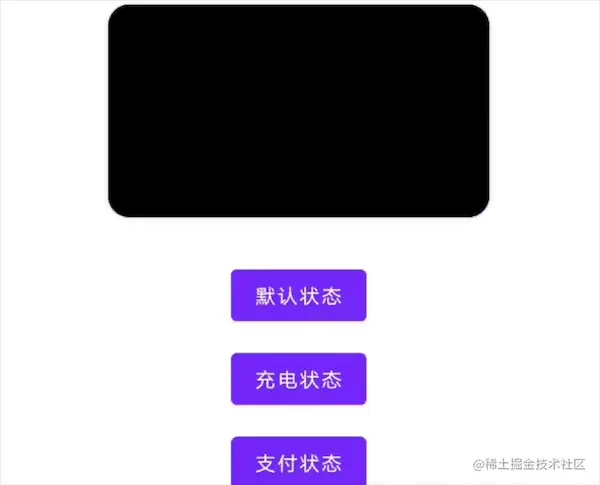## 多应用状态

``````val animateDpAsState by animateDpAsState(
targetValue = if (boxState is BoxState.MoreState) 105.dp else 70.dp,
animationSpec = spring(
dampingRatio = Spring.DampingRatioLowBouncy,
stiffness = Spring.StiffnessMediumLow
)
)
​
Box {
Box(
modifier = Modifier
.width(animateSizeAsState.width.dp)
.height(animateSizeAsState.height.dp)
.shadow(elevation = 3.dp, shape = RoundedCornerShape(15.dp))
.background(color = Color.Black),
)
Box(
modifier = Modifier
.size(30.dp)
.shadow(elevation = 3.dp, shape = RoundedCornerShape(15.dp))
.background(color = Color.Black)
)
}
``````

## 其他方案实现

``````val transition = updateTransition(targetState = boxState, label = "transition")
​
val boxHeight by transition.animateDp(label = "height", transitionSpec = boxSizeSpec()) {
boxState.height
}
val boxWidth by transition.animateDp(label = "width", transitionSpec = boxSizeSpec()) {
boxState.width
}
​
Box(
modifier = Modifier
.width(boxWidth)
.height(boxHeight)
.shadow(elevation = 3.dp, shape = RoundedCornerShape(15.dp))
.background(color = Color.Black),
)
``````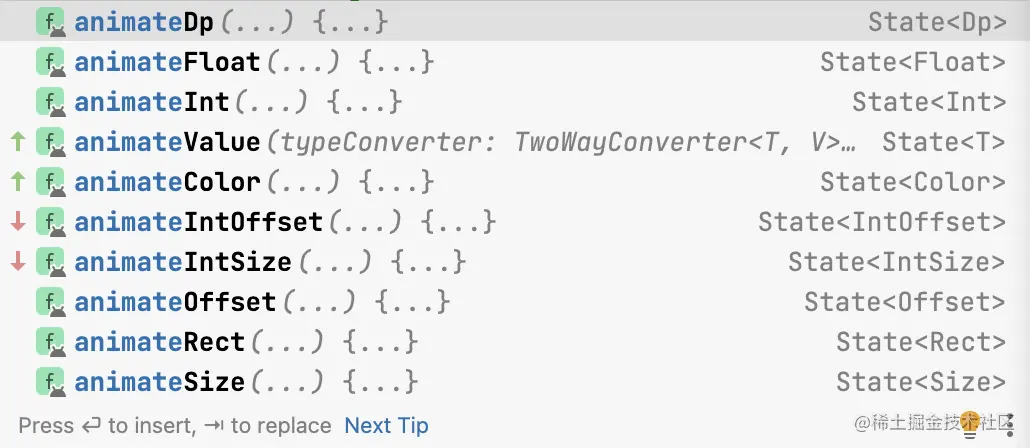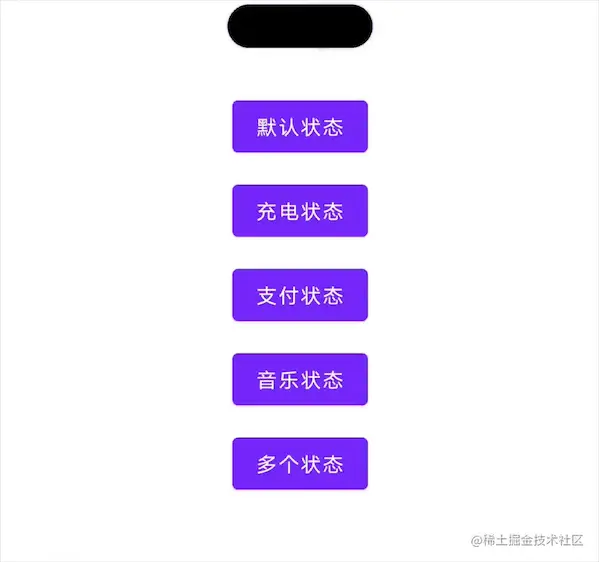## 多个应用切换优化

``````Canvas(modifier = Modifier.padding(start = 70.dp)) {
val path = Path()
val width = (animateFloatAsState + 30) * density
val x = animateFloatAsState * density
val p2x = density * 15f
val p2y = density * 25f
val p1x = density * 15f
val p1y = density * 5f
val p4x = width - 15f * density
val p4y = density * 30f
val p3x = width - 15f * density
val p3y = 0f
val c2x = (abs(p4x - p2x)) / 2
val c2y = density * 20f
val c1x = (abs(p3x - p1x)) / 2
val c1y = density * 10f
path.moveTo(p2x, p2y)
path.lineTo(p1x, p1y)
// 用二阶贝塞尔曲线画右边的曲线，参数的第一个点是上面的一个控制点
path.lineTo(p4x, p4y)
// 用二阶贝塞尔曲线画左边边的曲线，参数的第一个点是下面的一个控制点
​
if (animateFloatAsState == 35f) {
path.reset()
} else {
drawPath(
path = path, color = Color.Black,
style = Fill
)
}
​
path.addOval(Rect(x + 0f, 0f, x + density * 30f, density * 30f))
path.close()
drawPath(
path = path, color = Color.Black,
style = Fill
)
}
``````